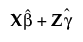Fitting Linear Models > Mixed Models > Conditional Model Inference > Actual by Conditional Predicted Plot
Publication date: 08/13/2020

##Actual by Conditional Predicted Plot

The Actual by Conditional Predicted plot appears by default. It provides a visual assessment of model fit that accounts for variation due to random effects. It plots the observed values of Y against the conditional predicted values of Y. These are the predicted values obtained if you select Save Columns > Conditional Prediction Formula.

Denote the linear mixed model by E[Y|γ] = Xβ + Zγ. Here β is the vector of fixed effect coefficients and γ is the vector of random effect coefficients. The conditional predictions are the predictions obtained from the model given by.